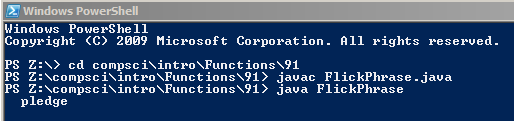# Assignemnt #91 and Flicker Phrase

## Code

``` ///name: ben borglin
///program name: Flicker Phrase
///file name: FlickPhrase.java
///period: 6
///date finished: 11/5/15

import java.util.Random;

public class FlickPhrase
{
public static void main( String[] args ) throws InterruptedException
{
Random rng = new Random();
int r;

for ( int i=0; i<100000; i++ )
{
r = 1 + rng.nextInt(5);
// Write five if statements here.
if (r == 1)
{
first();
}
if (r == 2)
{
second();
}
if (r == 3)
{
third();
}
if (r == 4)
{
fourth();
}
if (r == 5)
{
fifth();
}
}

System.out.println("I pledge allegiance to the flag.");

}

public static void first()
{
System.out.print("I                               \r");
}

public static void second()
{
System.out.print("  pledge                        \r");
}

public static void third()
{
System.out.print("         allegiance             \r");
}

public static void fourth()
{
System.out.print("                    to the      \r");
}

public static void fifth()
{
System.out.print("                           flag.\r");
}

}```